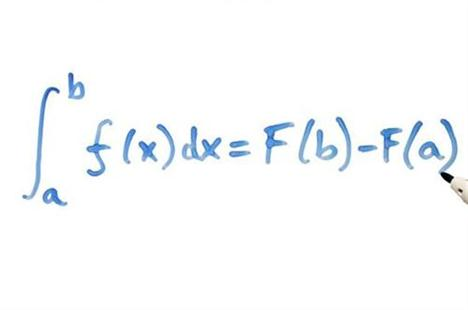Mar
19
2011

# Who Invented Calculus

The vast history of calculus has its history that goes back to 1820 BC. Egyptians had calculus but did not know the exact formulas, some of which are today considered invalid. Later on Archimedes developed the study of limits which was earlier studied by Greek mathematician Euxodus. The formulas for finding the areas of geometrical shapes were later developed in countries like China in 3rd century AD. The history of derivative came in 12th century when Bhaskara II from India developed the use of infinitesimal change. This was later known in modified terms as the Rolle’s teorem.

Issac Newton’s contribution to physics and mathematics is vast and calculus is one of them. He not just gave the chain and the product rule; he also focused his study on the use of higher derivatives. Gottfried Wilhelm Leibniz is a popular mathematician who made calculus as systematic as it is today. His contribution in spelling out the exact rules of calculus was as significant as Newton’s application of calculus in mathematics and physics. A debate on the title of the inventor of calculus went on for long. However both are considered as the inventors of calculus in own right as their approaches were much different.### Related Guides